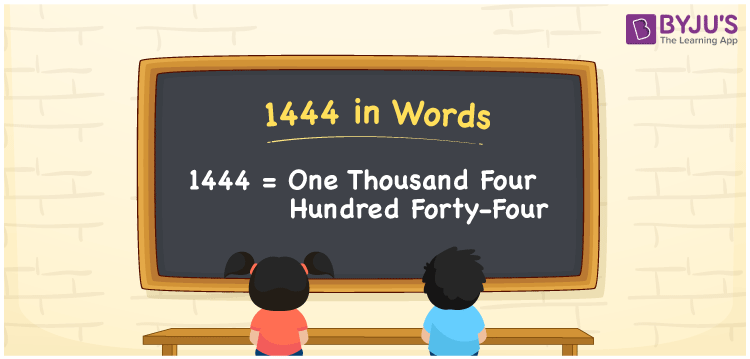# 1444 in words

1444 in words is written as One Thousand Four Hundred and Forty Four. 1444 represents the count or value. The article on Counting Numbers can give you an idea about count or counting. The number 1444 is used in expressions that relate to money, distance, length, year and others. Let us consider an example for 1444. ”In the year One Thousand Four Hundred and Forty Four, there was a fire accident at London’s Cathedral”.

 1444 in words One Thousand Four Hundred and Forty Four One Thousand Four Hundred and Forty Four in Numbers 1444

## 1444 in English Words## How to Write 1444 in Words?

We can convert 1444 to words using a place value chart. The number 1444 has 4 digits, so let’s make a chart that shows the place value up to 4 digits.

 Thousands Hundreds Tens Ones 1 4 4 4

Thus, we can write the expanded form as:

1 × Thousand + 4 × Hundred + 4 × Ten + 4 × One

= 1 × 1000 + 4 × 100 + 4 × 10 + 4 × 1

= 1444

= One Thousand Four Hundred and Forty Four.

1444 is the natural number that is succeeded by 1443 and preceded by 1445.

1444 in words – One Thousand Four Hundred and Forty Four.

Is 1444 an odd number? – No.

Is 1444 an even number? – Yes.

Is 1444 a perfect square number? – Yes. ( 38 × 38 )

Is 1444 a perfect cube number? – No.

Is 1444 a prime number? – No.

Is 1444 a composite number? – Yes.

## Solved Example

1. Write the number 1444 in expanded form

Solution: 1 × 1000 + 4 × 100 + 4 × 10 + 4 × 1

We can write 1444 = 1000 + 400 + 40 + 4

= 1 × 1000 + 4 × 100 + 4 × 10 + 4 × 1

## Frequently Asked Questions on 1444 in words

Q1

### How to write 1444 in words?

1444 in words is written as One Thousand Four Hundred and Forty Four.
Q2

### Is 1444 a perfect square number?

Yes. 1444 is a perfect square number. 38 multiplied by 38 is 1444.
Q3

### Is 1444 a prime number?

No. 1444 is not a prime number.